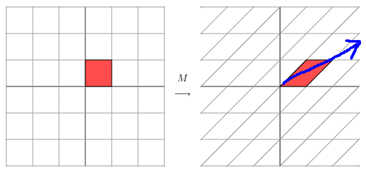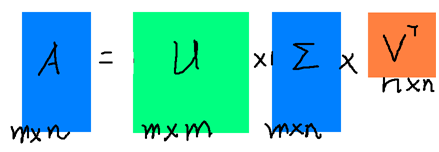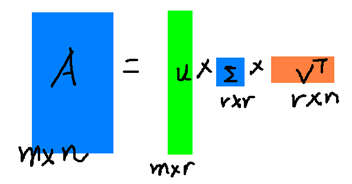# SVD 详解 与 spark实战

## 2.特征值、特征向量、特征值分解

http://blog.csdn.net/bitcarmanlee/article/details/52067985一文中，为大家解释了一个核心观点：矩阵是线性空间里的变换的描述。在有了这个认识的基础上，咱们接着往下。

N$N$ 维非零向量 x$x$N×N$N×N$ 的矩阵 A$A$ 的特征向量，当且仅当下式成立：Ax=λx$Ax = \lambda x$

A=QΣQ1

M=[xy]=[3xy]## 3.SVD分解

A=UΣVTAm×nUm×rΣr×rVr×n## 4.SVD分解的应用

SVD在实际中应用非常广泛，每个应用场景再单写一篇文章都没有问题。这里我们先不做过多的展开，先举两个最重要的方面。为了方便后面的描述，先把SVD的近似表达式再拎出来

Am×nUm×rΣr×rVr×n

## 5.spark中SVD分解的计算方法

spark里SVD是在MLlib包里的Dimensionality Reduction里(spark版本1.6，以下所有api与代码都是基于此版本)。文档里有SVD原理的简单描述。原理前面我们已经讲过，就不在重复了。重点看看里面的Performance:
We assume n$n$ is smaller than m$m$.The singular values and the right singular vectors are derived from the eigenvalues and the eigenvectors of the Gramian matrix ATA$A^TA$.The matrix storing the left singular vectors U$U$,is computed via matrix multiplication as U=A(VS1)$U = A(VS^{-1})$, if requested by the user via the computeU parameter. The actual method to use is determined automatically based on the computational cost:

If n$n$ is small (n$n$<100) or k$k$ is large compared with n$n$(k>n/2$k>n/2$),we compute the Gramian matrix first and then compute its top eigenvalues and eigenvectors locally on the driver. This requires a single pass with O(n2)$O(n^2)$ storage on each executor and on the driver, and O(n2k)$O(n^2k)$ time on the driver.

1.如果n$n$很小（n<100$n<100$），或者k$k$n$n$相比比较大（k>n/2$k > n/2$），那么先计算格莱姆矩阵ATA$A^TA$，再在spark的driver本地上计算其特征值与特征向量。这种方法需要在每个executor上O(n2)$O(n^2)$的存储空间，driver上O(n2)$O(n^2)$的存储空间，以及O(n2k)$O(n^2k)$的时间复杂度。
2.如果不是上面的第一种情况，那么先用分布式的方法计算(ATA)ν$(A^TA)\nu$在把结果传到ARPACK上用于后续再每个driver节点上计算ATA$A^TA$的前几个特征值与特征向量。这种方法需要O(k)$O(k)$的网络传输，每个executor上O(n)$O(n)$的存储，以及driver上的O(nk)$O(nk)$的存储。

## 6.spark SVD实战

http://blog.csdn.net/bitcarmanlee/article/details/51967323 spark集群搭建
http://blog.csdn.net/bitcarmanlee/article/details/52048976 spark本地开发环境搭建

import org.apache.spark.{SparkConf, SparkContext}
import org.apache.spark.mllib.linalg.{Matrix, SingularValueDecomposition, Vectors, Vector}
import org.apache.spark.mllib.linalg.distributed.RowMatrix

/**
* Created by lei.wang on 16/7/29.
*/

object SvdTest {

def main(args: Array[String]): Unit = {
val sc = new SparkContext(conf)
val data = Array(
Vectors.sparse(5,Seq((1,1.0),(3,7.0))),
Vectors.dense(2.0,0.0,3.0,4.0,5.0),
Vectors.dense(4.0,0.0,0.0,6.0,7.0))

val dataRDD = sc.parallelize(data,2)
val mat:RowMatrix = new RowMatrix(dataRDD)

val svd: SingularValueDecomposition[RowMatrix,Matrix] = mat.computeSVD(5,computeU = true)
val U:RowMatrix = svd.U //U矩阵
val s:Vector = svd.s //奇异值
val V:Matrix = svd.V //V矩阵

println(s)
}
}


...
The singular values is:
[13.029275535600473,5.368578733451684,2.5330498218813755,6.323166049206486E-8,2.0226934557075942E-8]
...©️2019 CSDN 皮肤主题: 编程工作室 设计师: CSDN官方博客